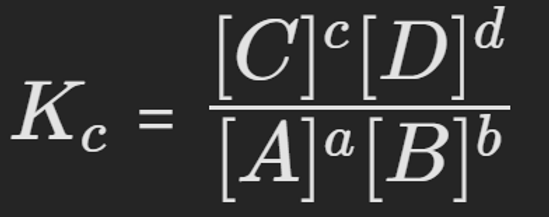top of page# Learning Objectives###### ​###### Le Chatelier's PrincipleIf a system is subject to change, the system will partially counteract the effect of the change by favouring the either the forward or reverse reaction.###### The Equilibrium Constant (K)General Equilibrium Equation

aA + bB cC + dD

Where the capitals = compound, and lower case = coefficient

The Equilibrium Constant (Kc)

• The equilibrium constant is used to demonstrate "the ratio of the ‘equilibrium product concentrations’ relative to the ‘equilibrium reactant concentrations’"

• In other words: The equilibirum constant shows the relative proportion of products and reactants once equilibrium is reached

• This applies to only Gases and Aqueous substances (do not include solids or liquids in the equation)

Equilibrium constant = Kc =([C]ᶜ[D]ᵈ) / ([A]ᵃ[B]ᵇ)

What Does The Equilibrium Constant Tell Us?

• If the Kc is approximately 1, there is a similar concentration of products and reactants

• If the Kc very large (>10⁴), equilibrium lies right, and there is a greater concentration of products

• If the Kc very small (<10⁴), equilibrium lies left, and there is a greater concentration of reactants###### Effect of Temperature on the K ConstantOnly temperature affects the K constant, effect temperature has on the K constant depends on the type of reaction.

• Increasing temperature favours the endothermic reaction. The system partially counteracts this increase by favouring the reaction which absorbs heat (the endothermic reaction)

• Decreasing temperature favours the exothermic reaction. The system partially counteracts the decrease by favouring the reaction which produces heat (the exothermic reaction)

• Decreasing temperature decreases the kinetic energy of all particles, reducing the frequency of collisions and decreasing reaction rate for both reactions. The decrease in rate is more profound for endothermic reaction as it has a higher activation energy, thus the reaction rate is faster for the exothermic reaction.###### What if the System is Not at Equilibirum?When the reaction is not at equilbrium, we are unable to use the K constant, instead we must rely on:

"The Reaction Quotient (Q)”

The reaction quotient shares the same equation with K, but instead provides the relative proportions at any given time, and can be used to show how a state of equilibrium can be reached.

By comparing Q to K we can deduce where the reaction is compared to the state of equilibrium:

• If Q = K, the system is at equilibrium

• If Q < K, System favours the forward reaction (equilibrium lies right)

• If Q > K, System favours the reverse reaction (equilibrium lies left)###### ​###### ​###### ​###### ​bottom of page